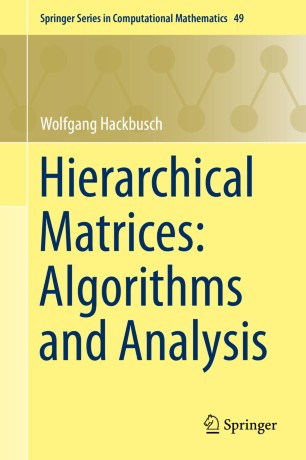# Hierarchical Matrices: Algorithms and Analysis

• Wolfgang HackbuschBook

Part of the Springer Series in Computational Mathematics book series (SSCM, volume 49)

1. Front Matter
Pages i-xxv
2. ### Introductory and Preparatory Topics

1. Front Matter
Pages 1-2
2. Wolfgang Hackbusch
Pages 3-24
3. Wolfgang Hackbusch
Pages 25-40
4. Wolfgang Hackbusch
Pages 41-52
5. Wolfgang Hackbusch
Pages 53-82
6. Wolfgang Hackbusch
Pages 83-116
3. ### $$\mathcal{H}$$ -Matrices and Their Arithmetic

1. Front Matter
Pages 117-118
2. Wolfgang Hackbusch
Pages 119-154
3. Wolfgang Hackbusch
Pages 155-202
4. Wolfgang Hackbusch
Pages 203-240
5. Wolfgang Hackbusch
Pages 241-275
4. ### Applications

1. Front Matter
Pages 277-278
2. Wolfgang Hackbusch
Pages 279-294
3. Wolfgang Hackbusch
Pages 295-319
4. Wolfgang Hackbusch
Pages 321-342
5. Wolfgang Hackbusch
Pages 343-354
6. Wolfgang Hackbusch
Pages 355-368
7. Wolfgang Hackbusch
Pages 369-378
8. Wolfgang Hackbusch
Pages 379-394
5. Back Matter
Pages 395-511

### Introduction

This self-contained monograph presents matrix algorithms and their analysis. The new technique enables not only the solution of linear systems but also the approximation of matrix functions, e.g., the matrix exponential. Other applications include the solution of matrix equations, e.g., the Lyapunov or Riccati equation. The required mathematical background can be found in the appendix.

The numerical treatment of fully populated large-scale matrices is usually rather costly. However, the technique of hierarchical matrices makes it possible to store matrices and to perform matrix operations approximately with almost linear cost and a controllable degree of approximation error. For important classes of matrices, the computational cost increases only logarithmically with the approximation error. The operations provided include the matrix inversion and LU decomposition.

Since large-scale linear algebra problems are standard in scientific computing, the subject of hierarchical matrices is of interest to scientists in computational mathematics, physics, chemistry and engineering.

### Keywords

efficient matrix algorithms matrix equations matrix functions matrix inversion and LU decomposition reconditioning

#### Authors and affiliations

• Wolfgang Hackbusch
• 1
1. 1.NaturwissenschaftenMPI für Mathematik in denLeipzigGermany

### Bibliographic information

Industry Sectors
Energy, Utilities & Environment
Engineering